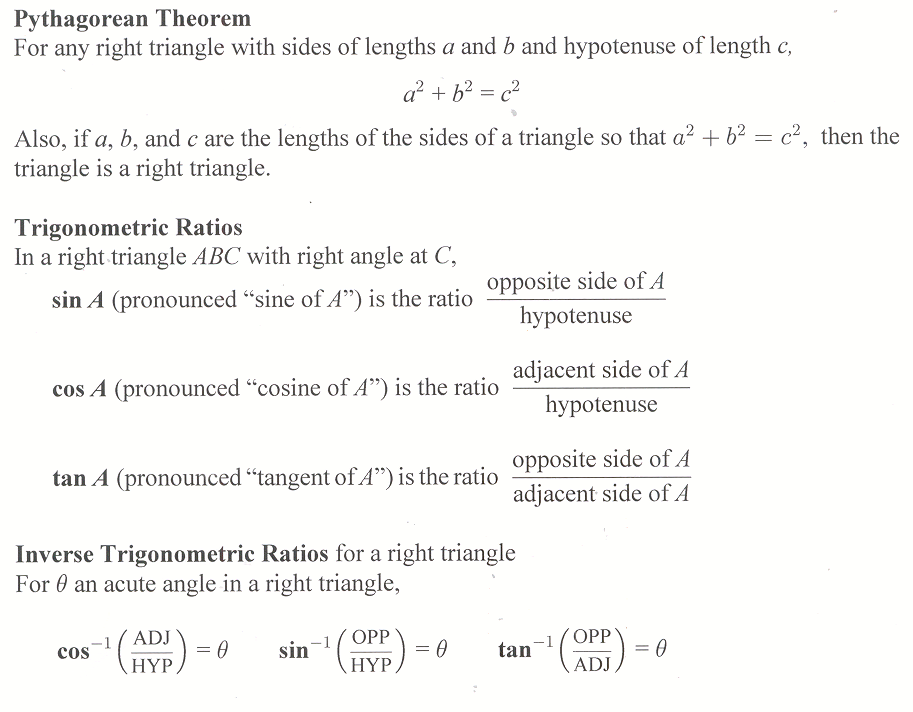## Homework Hints 7-5

[tippy title=”Problems 1-4″]Problems 1-4
These problems are designed to make sure you are familiar with the Pythagorean theorem as well as the definitions of sine, cosine, and tangent.[/tippy]

[tippy title=”Problem 5″]Problem 5
Use the Pythagorean theorem and Example 1.[/tippy]

[tippy See the whole entry

## 7.5 Outline

1. Pythagorean theorem
2. Trigonometric ratios
1. sine
2. cosine
3. tangent
3. Inverse trigonometric ratios
1. calculator calculating
2. inverse trigonometric ratios
1. inverse tangent
2. inverse cosine
3. inverse sine
4. Applications
1. angle of elevation
2. angle of depression

## 7.5 Essential IdeasSee the whole entry